Tuesday , 27 September 2022

# Set Theory Symbols

Set Theory Symbols – List of all set theory symbols and signs – meaning and examples.

Symbol Symbol Name Meaning / definition Example
{ } set a collection of elements A = {3,7,9,14}, B = {9,14,28}
A ∩ B intersection objects that belong to set A and set B A ∩ B = {9,14}
A ∪ B union objects that belong to set A or set B A ∪ B = {3,7,9,14,28}
A ⊆ B subset subset has fewer elements or equal to the set {9,14,28} ⊆ {9,14,28}
A ⊂ B proper subset / strict subset subset has fewer elements than the set {9,14} ⊂ {9,14,28}
A ⊄ B not subset left set not a subset of right set {9,66} ⊄ {9,14,28}
A ⊇ B superset set A has more elements or equal to the set B {9,14,28} ⊇ 9,14,28}
A ⊃ B proper superset / strict superset set A has more elements than set B {9,14,28} ⊃ {9,14}
A ⊅ B not superset set A is not a superset of set B {9,14,28} ⊅ {9,66}
2A power set all subsets of A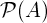power set all subsets of A
A = B equality both sets have the same members A={3,9,14}, B={3,9,14}, A=B
Ac complement all the objects that do not belong to set A
A \ B relative complement objects that belong to A and not to B A = {3,9,14}, B = {1,2,3}, A-B = {9,14}
A – B relative complement objects that belong to A and not to B A = {3,9,14}, B = {1,2,3}, A-B = {9,14}
A ∆ B symmetric difference objects that belong to A or B but not to their intersection A = {3,9,14}, B = {1,2,3}, A ∆ B = {1,2,9,14}
A ⊖ B symmetric difference objects that belong to A or B but not to their intersection A = {3,9,14}, B = {1,2,3}, A ⊖ B = {1,2,9,14}
a∈A element of set membership  A={3,9,14}, 3 ∈ A
x∉A not element of no set membership A={3,9,14}, 1 ∉ A
(a,b) ordered pair collection of 2 elements
A×B cartesian product set of all ordered pairs from A and B
|A| cardinality the number of elements of set A A={3,9,14}, |A|=3
#A cardinality the number of elements of set A A={3,9,14}, #A=3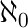aleph-null infinite cardinality of natural numbers set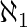aleph-one cardinality of countable ordinal numbers set
Ø empty set Ø = { } C = {Ø}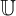universal set set of all possible values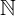0 natural numbers / whole numbers  set (with zero)0 = {0,1,2,3,4,…} 0 ∈01 natural numbers / whole numbers  set (without zero)1 = {1,2,3,4,5,…} 6 ∈1integer numbers set= {…-3,-2,-1,0,1,2,3,…} -6 ∈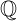rational numbers set= {x | x=a/ba,b} 2/6 ∈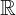real numbers set= {x | -∞ < x <∞} 6.343434 ∈complex numbers set= {z | z=a+bi, -∞<a<∞, -∞<b<∞} 6+2## World Tourism Day Information (27 Sept)

Since 1980, the United Nations World Tourism Organization has celebrated World Tourism Day (WTD) as …